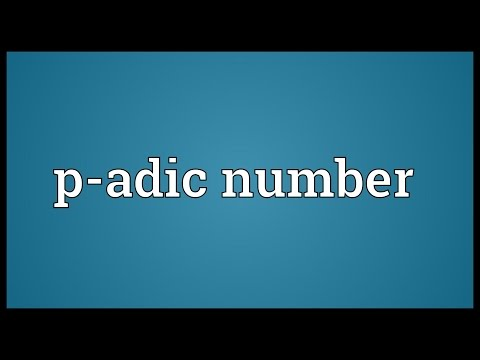Tip. You can look up words, expressions, names, titles...## Definition of the noun P-adic number

What does P-adic number mean as a name of something?

noun

1. [mathematics] an element of an extention of the field of rational numbers based on the divisibility of integers by a prime number p

## Explanation

p-adic number: In mathematics the p-adic number system for any prime number p extends the ordinary arithmetic of the rational numbers in a way different from the extension of the rational number system to the real and complex number systems. The extension is achieved by an alternative interpretation of the concept of "closeness" or absolute value. In particular, p-adic numbers have the interesting property that they are said to be close when their difference is divisible by a high power of p – the higher the power the closer they are. This property enables p-adic numbers to encode congruence information in a way that turns out to have powerful applications in number theory including, for example, in the famous proof of Fermat's Last Theorem by Andrew Wiles.

## Printed encyclopedias and other books with definitions for P-adic number

Click on a title to look inside that book (if available):

###Companion Encyclopedia of the History and Philosophy of the Mathematical Sciences (2002)

by Ivor Grattan-Guinness

Hensel and Hasse: p-adic numbers It was also this analogy between number fields and function fields that led Kronecker's pupil Kurt Hensel to introduce in 1897 the 'p-adic numbers', in connection with the determination of the precise prime power which divides the discriminant of an algebraic number field. A p-adic number of is a formal power series in the prime number p: oo ...

## Online dictionaries and encyclopedias with entries for P-adic number

Click on a label to prioritize search results according to that topic: